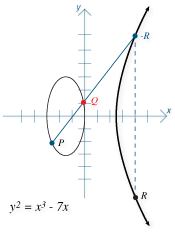The Internet - Math Matters - Maplesoft

# The Internet

Part of the growth of the internet has been due to mathematical techniques designed to maintain privacy and authenticity of information. Cryptography is the mathematical science behind the encryption schemes that do this.

The RSA (Rivest-Shamir-Adleman) algorithm is an example of a public key encryption algorithm that offers an elegantly simple way of storing and sharing the keys needed to unlock sensitive information. RSA is the main algorithm behind the security infrastructure that we use on the Web. The math in RSA centers around the difficulty in factoring large integers, that is, to defeat an RSA scheme you must be able to solve a virtually impossible factoring problem.

n = pq (p and q are distinct, typically large prime numbers)
m = (p-1)(p-1)

Choose e such that e is relatively prime to m, that is, no number other than 1 divides into e and m.

Find d such that de mod m = 1. If p and q are large, solving for d by breaking the key is virtually impossible.This number d is part of the secret (or private) key that unlocks hidden information. The number e is part of the public key that allows others to lock up information destined for specific people.

Another important public key algorithm uses elliptic curves over a finite field to supply the impossible problem. The advantage over RSA is that smaller numbers can be used with equal security. This is important for mobile devices where speed and space are issues.Julius Caesar
(Roman, 100 BC – 44 BC)
used encryption to communicate with his generals. Named after its first recorded user, the Caesar cipher shifts each letter of a message by a constant number of letters. The constant is the “key”.